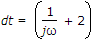# Electronics and Communication Engineering - Exam Questions Papers

6.

An air-filled rectangular waveguide has dimensions 8cm x 10cm . The wavelength of waveguide at frequency 3 GHz

 A. 25 cm B. 100 cm C. 16.13 cm D. 23.09 cm

Explanation:

λc = 2a = 2(10) = 20 cm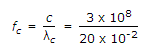= 1.5 GHz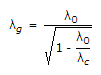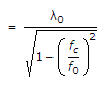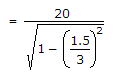λg = 23.09 cm.

7.

NMOS devices have __________ switching speeds and __________ on-state resistance; as compared with PMOS devices.

 A. slower, higher B. faster, lower C. faster, higher D. slower, lower

Explanation:

In NMOS devices, we have mobility of electrons than holes in PMOS; consequently higher conductivity and lower on-state resistance.

8.

What is the frequency of pulse at the points a, b, c in the circuit?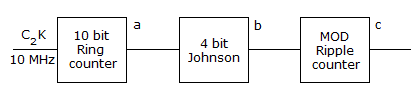A. 3 MHz B. 7.8 KHz C. 10 KHz D. 1 MHz

Explanation:

Ring counter is N : 1 divider.

Hence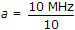⇒ 1 MHz

Johnson counter is 2N : 1 divider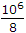Ripple counter 2N : 1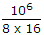= 7812.5 ∼ 7.8 kHz

Mod 10 = 4 FF ⇒ 24 = 16.

9.

A lossless transmission line with air dielectric is 6 m long. what is the phase constant?

Explanation: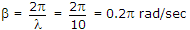.

10.

The impulse response h(t) of a linear invariant continuous time system is given by h(t) = exp (- 2t) u(t), where u(t) denotes the unit step function. The frequency response H(ω) of this system in terms of angular frequency ω is given by H(ω)

 A.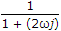B.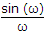C.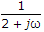D.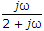H(ω) =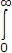e-2t e-jωt